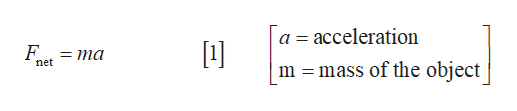You know the constant sum of the forces Feobject of mass m during t seconds, The object is at rest at thebeginning of the time interval.DP exerted on an

Question

Determine the acceleration during time period t.

determine the velocity at the end of time period t.

Determine the distance traveled during time period t.

express answers in variables Fnet, m, and thelp_outlineImage TranscriptioncloseYou know the constant sum of the forces Fe object of mass m during t seconds, The object is at rest at the beginning of the time interval. DP exerted on an fullscreen
Step 1

Given that the object is at rest at the beginning of time interval, and

Step 2

According to Newton’s second law, the net force on the object is,help_outlineImage Transcriptionclosea acceleration F = ma net mass of the object fullscreen
Step 3

Solve equation (1) for accele...

Want to see the full answer?

See Solution

Want to see this answer and more?

Our solutions are written by experts, many with advanced degrees, and available 24/7

See Solution
Tagged in

Newtons Laws of Motion